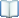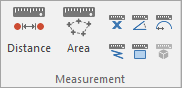#MeasurementMeasure Distance Measures the distance between two points. Measure Area Measures the area of a 'fence' polygon. Measure Position Measures a position relative to current axes. Measure Angle Measures the angle between two lines. Measure Geometry Radius Measures the radius of a curved line. Measure Geometry Length Adds up the lengths of the selected items. Measure Geometry Area Adds up the areas of the selected items. Measure Geometry Volume Measures the total volume of the selected items.